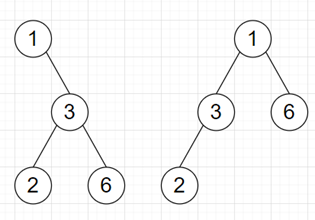# Program to check whether leaves sequences are same of two leaves or not in python

Suppose we have two binary trees; we have to check whether the sequence of leaves left-to-right in both trees are the same.

So, if the input is likethen the output will be True as the sequence is [2, 6] for both trees.

To solve this, we will follow these steps:

• c := a new list
• Define a function inorder() . This will take root, and c
• if c is null, then
• c := a new list
• if root is not null, then
• inorder(left of root, c)
• if left of root is null and right of root is null, then
• insert value of root at the end of c
• inorder(right of root, c)
• return c
• From the main method, do the following:
• if inorder(root0) is same as inorder(root1), then
• return True
• otherwise,
• return False

Let us see the following implementation to get better understanding:

## Example

Live Demo

class TreeNode:
def __init__(self, data, left = None, right = None):
self.val = data
self.left = left
self.right = right

class Solution:
c = []

def inorder(self, root, c=None):
if c is None:
c = []
if root:
self.inorder(root.left, c)
if not root.left and not root.right:
c.append(root.val)
self.inorder(root.right, c)
return c

def solve(self, root0, root1):
if self.inorder(root0) == self.inorder(root1):
return True
else:
return False

ob = Solution()
root1 = TreeNode(1)
root1.right = TreeNode(3)
root1.right.left = TreeNode(2)
root1.right.right = TreeNode(6)

root2 = TreeNode(1)
root2.left = TreeNode(3)
root2.right = TreeNode(6)
root2.left.left = TreeNode(2)
print(ob.solve(root1, root2))

## Input

root1 = TreeNode(1)
root1.right = TreeNode(3)
root1.right.left = TreeNode(2)root1.right.right = TreeNode(6)
root2 = TreeNode(1)
root2.left = TreeNode(3)root2.right = TreeNode(6)
root2.left.left = TreeNode(2)

## Output

True